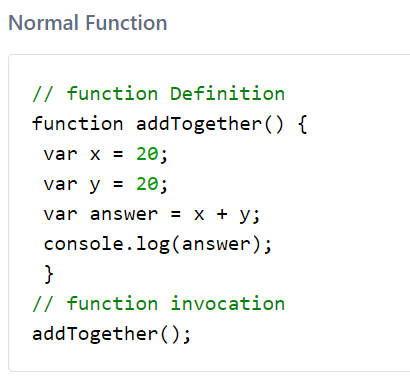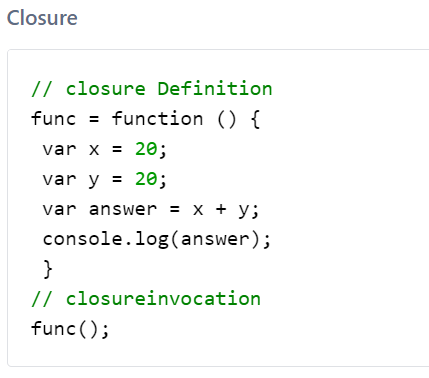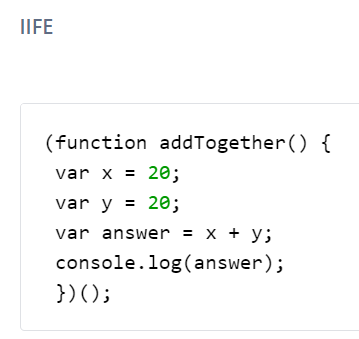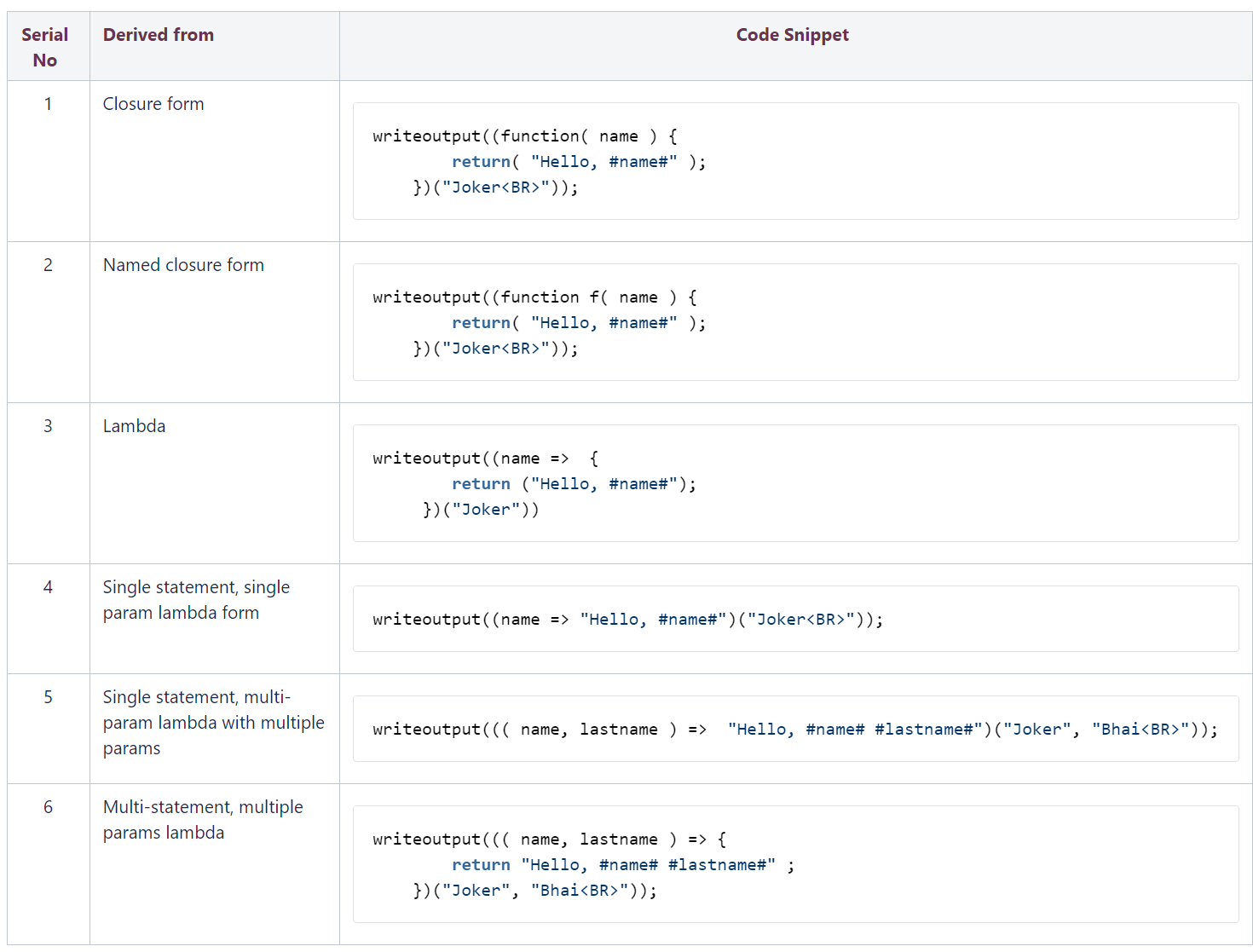An Immediately Invoked Function Expression is a good way at protecting the scope of your function and the variables within it. The term ‘scope’  basically just means where it can be accessed from. For example, if you were to add two numbers and log to console, you could do it use UDFs or closures as per CF 2018.With latest ColdFusion, we now support new way of doing the same thing, which we call Immediately Invoked Function Expressions (IIFEs). Above operation can be done in one statement.#### Characteristics

1. IIFEs to have there own scope i.e., the variables you declare in the Function Expression will not be available outside the function.
2. Similarly to other functions, IIFEs can also be named or anonymous, but even if an IIFE does have a name it is impossible to refer/invoke it.
3. IIFEs can also have parameters.
4. Once used an IIFE can’t be reused.

#### Various Forms of IIFE#### Usage Scenarios

##### Simple Statement
 (()=> {writeoutput(“simple statement test  passed
”)})();
##### Assignment Statement
 x = (()=> 2)()              // 2)()> writeoutput(x & “
”)
##### Multi-assignment Statement
 d = 3 a=b=c=d*(() => 5)() writeoutput(a)
##### Ternary Operator
 d = 2 a=b=c=d*(() => 5)() c = ((() => 55)() GT b ? a : (() => 55)() writeoutput(c)
##### Unary Operator
 writeoutput(-(() => 13)())
##### Parathensized expressions for precedence
 c = (((() => 15)()) – ((() => 13)()) ) * 2 writeoutput(c)
##### Return statement in UDF
 any function funky(mojo) { return      (() => mojo + 2)( ); } WriteOutput(funky(2) & “
”);
##### As a param to built-in / UDF methods
  ” more sleep!”)())> WRITEOUTPUT(myStringVar)
##### Switch Expression
 switch(( function () {return “orange”;})()) { case “apple”: WriteOutput(“I like Apples”); break; case “orange”: WriteOutput(“I like Oranges
”); break; default: WriteOutput(“I like fruit”);
##### IF Expression
 if(( function () {return “orange”;})() eq “orange”) writeoutput(“if test passed
”)
##### Recursive IIFE
 writeoutput(((param ) => param  + 15)((() => 13)()) )
##### Binary Operators
 writeoutput((() => 15)() – (() => 13)())
##### Loop
 for( i= 0 ; i<(() => 5)() ; i++ ) writeoutput(“df”)
##### Array Implicit Initialization
 a = [(()=> 2)(), (()=> 2)(), (()=> 2)()] writedump(a)
##### Struct Implicit Initialization
 b = { x = (()=> 2)() } writedump(##### Implicit Return Statement
 writedump((()=> (()=> 2)())())